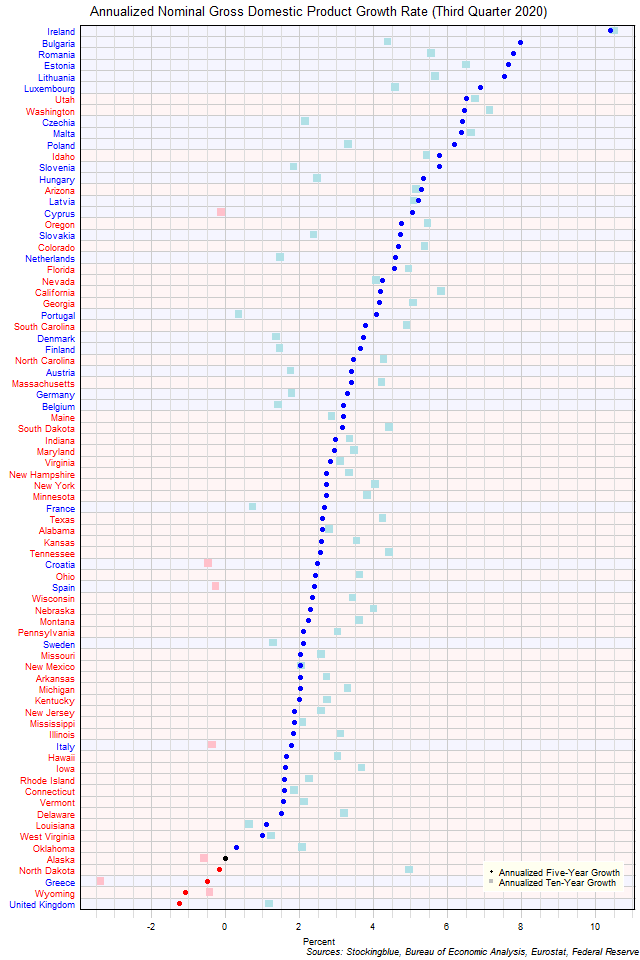# Long-Term EU and US GDP Growth Rate by State, Third Quarter 2020

Mar 4, 2021The chart above shows the annualized nominal gross domestic product (GDP) growth rate when the GDP is priced in US dollars in each EU and US state over the past five years as of the third quarter of 2020 and the growth rate over the past ten years.  Seven states had negative growth over the past ten years while four had negative growth over the past five.

## Findings

• The difference between the state with the largest annualized five-year growth rate, Ireland, and the state with the smallest, the United Kingdom, is 11.68 percentage points.
• The difference between the state with the largest annualized ten-year growth rate, Ireland, and the state with the smallest, Greece, is 13.88 percentage points.
• The median annualized five-year growth rate in the 28 EU states is 4.65% and the mean 4.60%.  The median annualized ten-year growth rate in the 28 EU states is 1.77% and the mean 2.48%.
• The median annualized five-year growth rate in the 50 US states is 2.49% and the mean 2.65%.  The median annualized ten-year growth rate in the 50 US states is 3.46% and the mean 3.50%.
• The median annualized five-year growth rate in the 78 EU and US states is 2.78% and the mean 3.35%.  The median annualized ten-year growth rate in the 78 EU and US states is 3.16% and the mean 3.13%.
• Of the 78 states, 31 grew faster over the past five years than they did over the past ten years (25 from the EU, 6 from the US), zero had the same annualized growth rate over both periods, and 47 grew slower over the past five years than they did over the past ten years (3 EU, 44 US).
• No EU drops in GDP growth rate are attributed to currency rate fluctuations.

## Caveats

• Data is from the third quarters of 2010, 2015, and 2020.
• The data is seasonally adjusted in current dollars.
• Euros are converted to dollars at an average exchange rate of 1.17 for the third quarter of 2020, 1.11 for the third quarter of 2015, and 1.29 for the third quarter of 2010 according to historic rates listed at the Federal Reserve (see source link below).
• US data comes in an annualized format which the EU does not, thus EU data is annualized by multiplying the quarterly figure by four.
• US growth rates may differ from those provided by the Bureau of Economic Analysis as the BEA's growth rates are based on chained dollars in conjunction with the chain index or the quality index for real GDP.  The growth rates listed here are based on nominal GDP.
• All figures are rounded to the nearest hundredth.

## Details

The United Kingdom had the worst performance over the past five years with an annualized growth rate of of -1.26%.  Ireland had the best performance with an annualized gain of 10.41%.

Over the past ten years, Greece had the worst performance with an annualized growth rate of -3.37% drop in GDP while Ireland had the best performance with an annualized growth rate of 10.51%.

North Dakota had the worst change in its growth rate between its annualized ten-year growth and its annualized five-year growth slowing its rate by 5.15 percentage points.  Cyprus had the best change in its growth rate between its annualized ten-year growth and its annualized five-year growth raising its rate by 5.17 percentage points.

Cyprus went up 55 spots in the growth rank from having the 72nd largest growth rate over the past ten years to the 17th largest growth rate over the past five years.  Conversely, North Dakota went from having the 15th highest growth rate over the past ten years to the 75th highest growth rate over the past five years.  Two states had annualized growth rates in the top five both over the past ten years and over the past five years: Ireland (highest ten-year, highest five-year) and Estonia (5th highest ten-year, 4th highest five-year).  Three states had growth rates in the bottom five both over both periods: Alaska (77th highest ten-year, 74th highest five-year), Greece (78th highest ten-year, 76th highest five-year), and Wyoming (75th highest ten-year, 77th highest five-year).

## Sources

Federal Reserve.  2021.  "Foreign Exchange Rates."  Accessed February 9, 2021.  https://www.federalreserve.gov/releases/g5/.

US Bureau of Economic Analysis.  2021.  "GDP by State."  Accessed February 5, 2021.  https://www.bea.gov/data/gdp/gdp-state.